Published: 21 October 2017

# Research on the near field sound source localization method for small snapshots

Qing Ji1
Jin Fang Cheng2
1, 2Department of Weaponry Engineering, Naval University of Engineering, Wuhan, China
Corresponding Author:
Qing Ji
Views 23

#### Abstract

The performance of standard MVDR algorithm would be decreased sharply when the sampled data is small. In this paper, vector MVDR algorithm and the diagonal loading are proposed to improve the robustness. Firstly, the sample date covariance matrix is whitened to obtain more uniform noise, and then use the standard deviation of covariance matrix as the loading amount to complete diagonal loading. Finally, the effectiveness and robustness of the proposed algorithm is verified by simulation. Simulation results reveal that it has higher positioning accuracy with small sample, with the increase of SNR and snapshots, the estimation’s RMSE of target location decreases continuously.

## 1. Introduction

Unmanned underwater vehicle (UUV) has become the important equipment for all countries, especially with the development of energy and control technology. UUVs are often able to get close to minefield and complete anti mine warfare, have become the serious threat to the safety of mine. Furthermore, because the low radiation noise of UUVs and the complexity of marine environment, the effective snapshots collected by detection systems are very little. For the mine, it is very important to improve the localization ability of near field target with the finite sample date [1, 2].

In 1969 Capon proposed the minimum variance distortionless beamforming (MVDR) algorithm, which is a high resolution spatial spectrum method . When the number of samples is small, the sample covariance matrix will be an ill-conditioned matrix and the localization performance of MVDR algorithm will greatly reduce. Carlson proposed diagonal loading technique to correct the covariance matrix, improved the stability of the MVDR algorithm . Later, many improved robust adaptive beamforming has been put forward . This paper takes the target positioning problem in mine’s near field as the research background. A vector hydrophone array is used for the receiving sensors. The diagonal loading and MVDR algorithm are applied to the near-field acoustic source localization with small sample, so as to improve the survival ability of mines.

## 2. The measurement model

It is assumed that the detection unit of mine is a uniform linear array consisting of $M$ three-dimensional vector hydrophones. Vector hydrophones receive the radiated noise of $N$ narrowband acoustic targets in the near field region of mine. The space positions of the array and target are shown in Fig. 1.

The acoustic pressure signal received by the vector hydrophones can be written in matrix form:

1
$\mathbf{P}={\mathbf{A}}_{p}\mathbf{s}\left(t\right)+{\mathbf{n}}_{p}\left(t\right),$

where, ${\mathbf{A}}_{p}$ is $M×N$-dimensional matrix, near field manifold matrix of scalar sound pressure array, and can be written as ${\mathbf{A}}_{p}=\left[\mathbf{a}\left({\theta }_{11},{\alpha }_{11},{r}_{11}\right),\mathbf{a}\left({\theta }_{21},{\alpha }_{21},{r}_{21}\right),...,\mathbf{a}\left({\theta }_{N1},{\alpha }_{N1},{r}_{N1}\right)\right]\text{,}$ where $\mathbf{a}\left({\theta }_{n1},{\alpha }_{n1},{r}_{n1}\right)={\left[1,\frac{{r}_{n1}}{{r}_{n2}}{e}^{\left(j{k}_{n}\left({r}_{n1}-{r}_{n2}\right)},...,\frac{{r}_{n1}}{{r}_{nM}}{e}^{\left(j{k}_{n}\left({r}_{n1}-{{r}_{n}}_{M}\right)}\right]}^{T}$.

In the near field, the relationship between the acoustic pressure and the vibration velocity received by a single vector hydrophone is no longer completely correlative. According to the Euler equation, the relationship between the vibration velocity ${v}_{mx}$, ${v}_{my}$, ${v}_{mz}$ and the acoustic pressure ${p}_{m}$ can be expressed by following formula:

2
$\left[\begin{array}{l}{v}_{mx}\\ \begin{array}{l}{v}_{my}\\ {v}_{mz}\end{array}\end{array}\right]=\frac{{p}_{m}}{{Z}_{nm}}\left[\begin{array}{c}\mathrm{s}\mathrm{i}\mathrm{n}{\alpha }_{nm}\mathrm{c}\mathrm{o}\mathrm{s}{\theta }_{nm}\\ \mathrm{s}\mathrm{i}\mathrm{n}{\alpha }_{nm}\mathrm{s}\mathrm{i}\mathrm{n}{\theta }_{nm}\\ \mathrm{c}\mathrm{o}\mathrm{s}{\alpha }_{nm}\end{array}\right]+\left[\begin{array}{l}{n}_{mx}\\ \begin{array}{l}{n}_{my}\\ {n}_{mz}\end{array}\end{array}\right]=\frac{{p}_{m}}{{Z}_{nm}}{\mathbf{u}}_{nm}+\left[{n}_{mx},{n}_{my},{n}_{mz}{\right]}^{T},$

where, ${n}_{mx}$, ${n}_{my}$, ${n}_{mz}$ are respectively the noises of the $m$th vector hydrophone’s vibration velocity channel. Power satisfy the relation: ${\sigma }_{p}^{2}/{\sigma }_{vx}^{2}=3$, ${\sigma }_{vx}^{2}={\sigma }_{vy}^{2}={\sigma }_{vz}^{2}$. ${Z}_{nm}$ is acoustic impedance in the near field, can be computed by:

3
${Z}_{nm}={\left(1-j\frac{{\lambda }_{n}}{2\pi {r}_{nm}}\right)}^{-1}.$

Fig. 1Positions of target and array in near field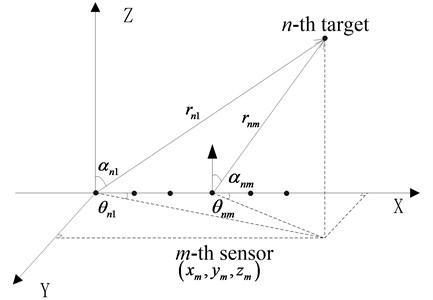To sum up, $\mathbf{X}\left(t\right)$ is $4M×1$-dimensional matrix, the data vector received by the vector hydrophone array can be written in following formula according to different hydrophone outputs:

4
$\mathbf{x}\left(t\right)={\left[{p}_{1}\left(t\right),{v}_{1x}\left(t\right),{v}_{1y}\left(t\right),{v}_{1z}\left(t\right),...,{p}_{M}\left(t\right),{v}_{Mx}\left(t\right),{v}_{My}\left(t\right),{v}_{Mz}\left(t\right)\right]}^{T}+\mathbf{n}\left(t\right)$
$=\left({1}_{4×1}\otimes {\mathbf{A}}_{p}\right)\odot \mathbf{U}\mathbf{s}\left(t\right)+\mathbf{n}\left(t\right)=\mathbb{C}\mathbf{s}\left(t\right)+\mathbf{n}\left(t\right).$

Among that, $\otimes$, $\odot$ respectively represent Kronecker product and Hadamard product. ${1}_{4×1}$, 4×1-dimension matrix, all the elements are 1. $\mathbb{C}\left(\theta ,\alpha ,\mathbf{r}\right)$ is $4M×N$-dimensional matrix, near field vector manifold matrix, and can be written as:

$\mathbb{C}\left(\mathbf{\theta },\mathbf{\alpha },\mathbf{r}\right)=\left[\mathbf{c}\left({\theta }_{11},{\alpha }_{11},{r}_{11}\right),...,\mathbf{c}\left({\theta }_{N1},{\alpha }_{N1},{r}_{N1}\right)\right].$

$\mathbf{U}$ is $4M×N$-dimensional matrix, correlation coefficient matrix of sound pressure and vibration velocity, the $\left(m,n\right)$-th partitioned matrix can be calculated: $\mathbf{U}\left(m,n\right)={\left[1,\frac{{{\mathrm{u}}^{T}}_{nm}}{{Z}_{nm}}\right]}^{T}$.

So, in this paper, the near field beamforming algorithm is used to estimate the parameters ${\theta }_{11}$, ${\alpha }_{11}$, ${r}_{11}$ of the acoustic source by vector near field measurement model, so as to achieve the target location and improve the survival ability of the mine.

## 3. The proposed algorithm

### 3.1. Near field vector MVDR

In order to break the poor resolution of the conventional beamforming, Capon proposed the MVDR beamformer, the design of the weight vector can be expressed as:

5
$\mathrm{m}\mathrm{i}\mathrm{n}\left\{\mathbf{w}\left(\theta ,\alpha ,r{\right)}^{H}\mathbf{R}\mathbf{w}\left(\theta ,\alpha ,r\right)\right\}\mathrm{}\mathrm{}\mathrm{}\mathrm{s}.\mathrm{t}.\mathrm{}\mathrm{}\mathrm{}\mathrm{}\mathbf{w}\left(\theta ,\alpha ,r\right)\mathbf{c}\left({\theta }_{s},{\alpha }_{s},{r}_{s}\right)=1.$

Using the Lagrange method, the optimal weight vector is obtained:

6
${\mathbf{w}}_{opt}\left({\theta }_{s},{\alpha }_{s},{r}_{s}\right)=\frac{{\mathbf{R}}^{-1}\mathbf{c}\left({\theta }_{s},{\alpha }_{s},{{r}_{s}}_{s}\right)}{{\mathbf{c}}^{H}\left({\theta }_{s},{\alpha }_{s},{r}_{s}\right){\mathbf{R}}^{-1}\mathbf{c}\left({\theta }_{s},{\alpha }_{s},{r}_{s}\right)}=\upsilon {\mathbf{R}}^{-1}\mathbf{c}\left({\theta }_{s},{\alpha }_{s},{r}_{s}\right),$

where, $\upsilon$ is a constant coefficient. The output spatial power spectrums function of the near field vector MVDR beamformer is:

7
${P}_{MVDR}\left({\theta }_{s},{\alpha }_{s},{r}_{s}\right)={{\mathbf{w}}^{H}}_{opt}{\mathbf{R}}^{-1}{\mathbf{w}}_{opt}=1/\left(\mathbf{c}\left({\theta }_{s},{\alpha }_{s},{r}_{s}{\right)}^{H}{\mathbf{R}}^{-1}\mathbf{c}\left({\theta }_{s},{\alpha }_{s},{r}_{s}\right)\right).$

$\mathbf{R}$ is the covariance matrix of sample signals, usually estimated by limited snapshots data, which are collected by the detection unit:

8
$\mathbf{R}=\frac{1}{{N}_{t}}\sum _{1}^{{N}_{t}}\mathbf{x}\left(t\right){\mathbf{x}}^{H}\left(t\right).$

Obviously, there is a direct relationship between the covariance matrix and data snapshots. Through eigen decomposition and inversion, we can obtain:

9
${\mathbf{R}}^{-1}=\mathbf{Ε}{\mathbf{\Lambda }}^{-1}{\mathbf{E}}^{H}=\sum _{m=1}^{M}\frac{1}{{\lambda }_{m}}{\mathbf{e}}_{m}{{\mathbf{e}}^{H}}_{m}=\frac{1}{{\lambda }_{\mathrm{m}\mathrm{i}\mathrm{n}}}\left(\mathbf{I}-\sum _{1}^{M}\frac{{\lambda }_{m}-{\lambda }_{\mathrm{m}\mathrm{i}\mathrm{n}}}{{\lambda }_{m}}{\mathbf{e}}_{m}{{\mathbf{e}}^{H}}_{m}\right).$

Descending order of eigenvalues, ${\lambda }_{1}\ge {\lambda }_{2}\ge \cdots {\lambda }_{N}\ge {\lambda }_{N+1}\cdots \ge {\lambda }_{M}$. ${\lambda }_{1},{\lambda }_{2},\dots ,{\lambda }_{N}$ are large eigenvalue corresponding to signals, generally far greater than the small eigenvalues corresponding to noise ${\lambda }_{N+1},\dots ,{\lambda }_{M}$. ${\lambda }_{\mathrm{m}\mathrm{i}\mathrm{n}}$, the minimum eigenvalue, is general the environment noise power. ${\mathbf{e}}_{m}$, the eigenvectors corresponding to eigenvalues, $\mathbf{Ε}=\left[{\mathbf{e}}_{1,}{\mathbf{e}}_{2},\dots ,{\mathbf{e}}_{M}\right]$.

The eigenvectors are substituted in Eq. (6) and ignoring the constant coefficient, the weight is:

10
${\mathbf{w}}_{opt}\left({\theta }_{s},{\alpha }_{s},{r}_{s}\right)=\left(\mathbf{I}-\sum _{m=1}^{N}\frac{{\lambda }_{m}-{\lambda }_{\mathrm{m}\mathrm{i}\mathrm{n}}}{{\lambda }_{m}}{\mathbf{e}}_{m}{{\mathbf{e}}^{H}}_{m}-\sum _{m=N+1}^{M}\frac{{\lambda }_{m}-{\lambda }_{\mathrm{m}\mathrm{i}\mathrm{n}}}{{\lambda }_{m}}{\mathbf{e}}_{m}{{\mathbf{e}}^{H}}_{m}\right)\mathbf{c}\left({\theta }_{s},{\alpha }_{s},{r}_{s}\right).$

Through analysis, we know that when the snapshot number is large enough, the estimation error of noise covariance matrix does not exist, all the small eigenvalues corresponding to noise can be written as ${\lambda }_{N+1}={\lambda }_{N+2}=\cdots ={\lambda }_{M}={\lambda }_{\mathrm{m}\mathrm{i}\mathrm{n}}$. Since the eigenvectors of the noise are orthogonal to the eigenvectors of the desired signal, the third term in the upper formula is zero. Conversely, the number of snapshots is small, ${\lambda }_{N+1}\ne {\lambda }_{N+2}\cdots \ne {\lambda }_{M}\ne {\lambda }_{\mathrm{m}\mathrm{i}\mathrm{n}}$. The eigenvalues stochastic fluctuation is diffusion, which makes the beam response of the noise subspace seriously affect the beam output of MVDR. Therefore, the standard MVDR is very sensitive with the number of snapshots, it is necessary to improve its robustness in the small samples number.

In isotropic environment noise, the noise power of sound pressure channel is not equal to that of the vibration velocity channel. There is a fixed proportional relationship: ${\sigma }_{p}^{2}/{\sigma }_{vx}^{2}=\text{3,}$${\sigma }_{vx}^{2}={\sigma }_{vy}^{2}={\sigma }_{vz}^{2}$. Ideally, the small eigenvalue of covariance matrix is inherently inhomogeneous. In this paper, we propose a preprocessing method for this problem. Firstly, the covariance matrix is whitened:

11
$\mathbf{B}\mathbf{R}{\mathbf{B}}^{H}=\mathbf{B}\mathbb{C}{\mathbf{R}}_{s}{\mathbb{C}}^{H}{\mathbf{B}}^{H}+{\sigma }_{p}^{2}\mathbf{I},$

where, the preprocessing matrix $\mathbf{B}=\mathbf{I}\otimes \mathrm{d}\mathrm{i}\mathrm{a}\mathrm{g}\left[1,{3}_{}^{1/2},{3}_{}^{1/2},{3}_{}^{1/2}\right]$. It can be seen from the Eq. (11) that the noise covariance matrix has been processed uniformly. For easy to explain, the covariance matrix after pretreatment, is still denoted as $\mathbf{R}$.

The diagonal loading technique adds a loading value $\xi$ to the diagonal elements of the covariance matrix, reduces the diffusion degree of noise eigenvalues, can improve the robustness of MVDR algorithm. After diagonal loading, the new covariance matrix is obtained:

12
${\mathbf{R}}_{DL}=\mathbf{R}+\xi \mathbf{I}=\mathbf{Ε}\left(\mathbf{\Lambda }+\xi \mathbf{I}{\right)}^{-1}{\mathbf{E}}^{H}.$

The Eq. (12) is substituted in Eq. (10) to obtain the weight vector after diagonal loading:

13
${\mathbf{w}}_{DL}\left({\theta }_{s},{\alpha }_{s},{r}_{s}\right)=\left(\mathbf{I}-\sum _{m=1}^{N}\frac{{\lambda }_{m}-{\lambda }_{\mathrm{m}\mathrm{i}\mathrm{n}}}{{\lambda }_{m}+\xi }{\mathbf{e}}_{m}{{\mathbf{e}}^{H}}_{m}-\sum _{m=N+1}^{M}\frac{{\lambda }_{m}-{\lambda }_{\mathrm{m}\mathrm{i}\mathrm{n}}}{{\lambda }_{m}+\xi }{\mathbf{e}}_{m}{{\mathbf{e}}^{H}}_{m}\right)\mathbf{c}\left({\theta }_{s},{\alpha }_{s},{r}_{s}\right).$

Generally, the loading value is larger than the noise eigenvalues, while less than large eigenvalues of the signal. At this time, $\frac{{\lambda }_{m}-{\lambda }_{\mathrm{m}\mathrm{i}\mathrm{n}}}{{\lambda }_{m}+\xi }\ll \frac{{\lambda }_{m}-{\lambda }_{\mathrm{m}\mathrm{i}\mathrm{n}}}{{\lambda }_{m}}$ when $N+1\le m\le M$. So, the fluctuation caused by the noise subspace will be reduced after diagonal loading, thus improving the robustness of the MVDR algorithm. Meanwhile, the beam in the signal subspace has little effect. It has been proved in the literature that when the standard deviation of covariance matrix is used as loading value, it is easy to realize, and without prior knowledge, it has the ability to adaptively adjust loading value .

In this paper, adaptive diagonal loading technique and vector hydrophones are used to locate small targets in the near field of mine, improve the robustness of the MVDR algorithm with small sample.

## 4. Simulation calculation

In this section, the positioning capability of algorithm will be verified through simulations. At the same time, the positioning performance changed with signal-to-noise ratio (SNR) and the number of snapshots will be analyzed. The spatial spectrum of search space can be calculated by MVDR and the diagonal loading MVDR after pretreatment (denoted as PMVDR in this paper). The coordinate of the spatial spectrum’s maximum will be regarded as the estimated value of target’s azimuth, pitch and range. A uniform linear array composed of 7 vector hydrophones is used in all simulations. The distance among elements is 1 meter. The target is a single frequency narrow-band sound source with a center frequency of $f=$1000 Hz and target location is ($\theta =$40°, $\alpha =$ 45°, $r=$ 30 m).

### 4.1. Simulation 1: analysis of positioning capability with small sample

This paper is mainly aimed at near-field acoustic source localization under small snapshots data, so set the number of snapshots is 5. The noise with SNR = 5 dB is added. The number of Monte Carlo experiments is 50. The spatial spectrum of search space will be obtained by vector MVDR and PMVDR algorithm. Furthermore, the distribution of the target coordinate is obtained, shown in Fig. 2.

In Fig. 2, the azimuth, elevation and range calculated by standard MVDR can fluctuate at the true value, but they disperse widely. The results are not stable. That is to say that the standard MVDR algorithm is sensitive with the number of snapshots, also verified the theory in the front. In comparison, the results calculated by PMVDR can focus on the true value, and disperse significantly smaller. The proposed MVDR algorithm, which was dealt with noise whitening pretreatment and diagonal loading, can improve the positioning accuracy of near field target with small snapshots.

Fig. 2The distribution of location parameters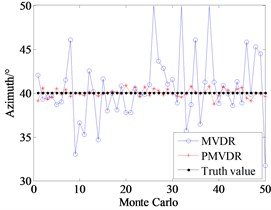a) Azimuth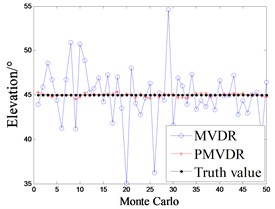b) Elevation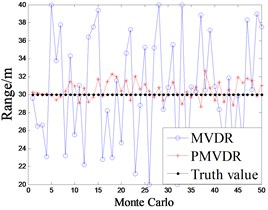c) Range

### 4.2. Simulation 2: the localization performance varies with SNR

The SNR changes from -10dB to 20dB, and the number of snapshots is 5. The root mean square error (RMSE) and CRB of estimated value can be achieved by 100 Monte-Carlo simulation experiments. The performance curves are shown in Fig. 3.

As shown in Fig. 3, it can be seen that the estimates’ RMSE of the MVDR algorithm has not been improved with the increase of SNR. The RMSE obtained by PMVDR algorithm and the CRB decrease with the increase of SNR, and the RMSE curve is constantly closed to CRB. It is proved that the performance of PMVDR algorithm has been improved with the increase of SNR and tends to CRB.

Fig. 3The positioning performance curve vs SNR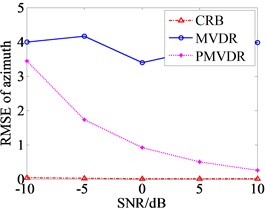a) Azimuth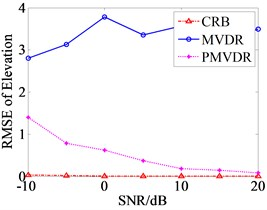b) Elevation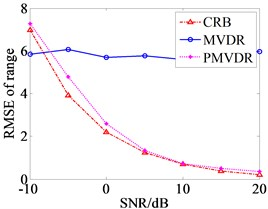c) Range

### 4.3. Simulation 3: The localization performance varies with snapshots

The algorithm performance changes with the number of snapshots, the snapshots number increased from 5 to 605, increased step 100. 100 simulations are performed to compute the RMSE and CRB, the performance curves are shown in Fig. 4.

The curves in Fig. 4 show that estimation error of MVDR algorithm is much larger than that of PMVDR and CRB when the snapshot number is small. With the increase of snapshots, the estimation error of MVDR algorithm decreases rapidly. It indicates that MVDR algorithm is very sensitive and unstable with small sample data. At the same time, the estimation error of PMVDR and CRB continue to decline. The performance of MVDR and PMVDR algorithm are not different when the snapshot number is large enough.

Fig. 4The positioning performance curve vs snapshots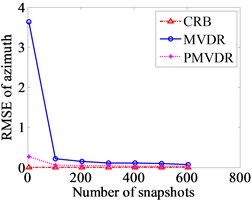a) Azimuth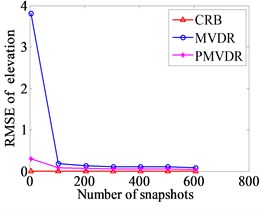b) Elevation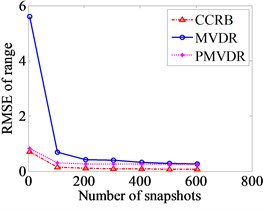c) Range

## 5. Conclusions

The poor performance of MVDR algorithm with small sampled data is studied in this paper. The vector hydrophone and the diagonal loading technique after preprocessing are proposed to improve the positioning performance of MVDR algorithm. Finally, simulation results demonstrate the effectiveness of proposed algorithm. With the small sample data, the simulation results show that the PMVDR algorithm can locate the near field target more accurately than the MVDR algorithm. With the increase of SNR and snapshots, the RMSE of estimation results continue to decrease, the positioning performance improved gradually. It can provide a new idea for target location of mine near field.